## Fluid Mechanics and Hydraulic Machinery Miscellaneous

#### Fluid Mechanics and Hydraulic Machinery

1. At the inlet of an axial impulse turbine rotor, the blade linear speed is 25 m/s, the magnitude of absolute velocity is 100 m/s and the angle between them is 25°. The relative velocity and the axial component of velocity remain the same between the inlet and outlet of the blades. The blade inlet and outlet velocity triangles are shown in figure. Assuming no losses, the specific work (in J/kg) is _______.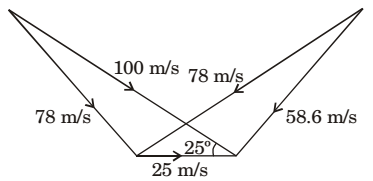1.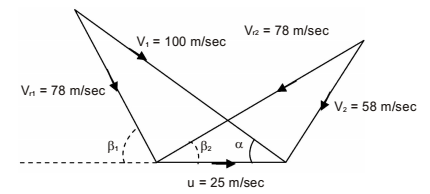Given α = 25º
Vr1 sin β1 =V1 sinα
∴ 78 sin β1 = 100 sin 25º
∴ β1 = 32.81º
∵ Vr1 = Vr2
∴ β1 = β2 = 32.81º
Now ∴ ∆Vw = Vr1 cosβ1 + Vr2 cosβ2
= 2Vr1 cosβ1
= 2 × 78 × cos32.81°
∴ ∆Vw = 131.1136 m/sec
∴ Sp. power = ∆Vw . u = 131.1136 × 25
= 3277.84 J/kg.

##### Correct Option: AGiven α = 25º
Vr1 sin β1 =V1 sinα
∴ 78 sin β1 = 100 sin 25º
∴ β1 = 32.81º
∵ Vr1 = Vr2
∴ β1 = β2 = 32.81º
Now ∴ ∆Vw = Vr1 cosβ1 + Vr2 cosβ2
= 2Vr1 cosβ1
= 2 × 78 × cos32.81°
∴ ∆Vw = 131.1136 m/sec
∴ Sp. power = ∆Vw . u = 131.1136 × 25
= 3277.84 J/kg.

1. A siphon draws water from a reservoir and discharge it out at atmospheric pressure.
Assuming ideal fluid and the reservoir is large, the velocity at point Pin the siphon tube is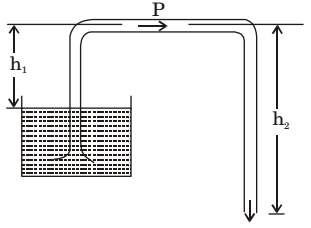1. By energy conservation, velocity at point
Q = √2g(h2 - h1)
As there is a continuous and uniform flow, so velocity of liquid at point Q and P is same.Vp =√2g(h2 - h1)

##### Correct Option: B

By energy conservation, velocity at point
Q = √2g(h2 - h1)
As there is a continuous and uniform flow, so velocity of liquid at point Q and P is same.Vp =√2g(h2 - h1)

1. Consider steady flow of an incompressible fluid through two long and straight pipes of diameters d1, and d2 arranged in series. Both pipes are of equal length and the flow is turbulent in both pipes. The friction factor for turbulent flow though pipes is of the for, f = K(Re)–n, Where K and n are known positive constants and Re is the Reynolds number. Neglecting minor losses, the ratio of the frictional pressure drop in pipe
 1 to that in pipe 2.∆P1, is given by ∆P2

1.  hf1 = f1IQ² × 12d25 hf2 12d15 f2IQ²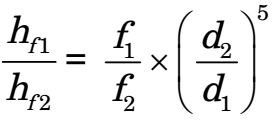f∝ Re-n
 f ∝ρVd-n μ

 f ∝ρQd-n π / d²μ

f ∝ dn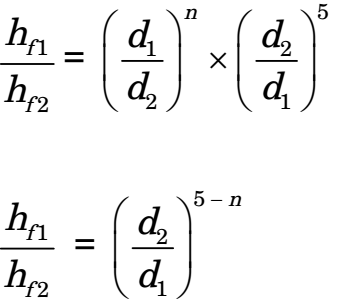##### Correct Option: A

 hf1 = f1IQ² × 12d25 hf2 12d15 f2IQ²f∝ Re-n
 f ∝ρVd-n μ

 f ∝ρQd-n π / d²μ

f ∝ dn1. A channel of width 450 mm branches into two sub-channels having width 300 mm and 200 mm as shown in figure. If the volumetric flow rate (taking unit depth) of an in compressible flow through the main channel is 0.9 m3/s and the velocity in the sub-channel of width 200 mm is 3 m/s, the velocity in the sub-channel of width 300 mm is ________m/s.
Assume both inlet and outlet to be at the same elevation.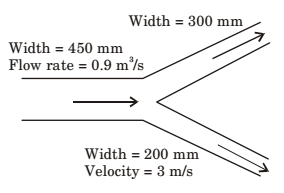1.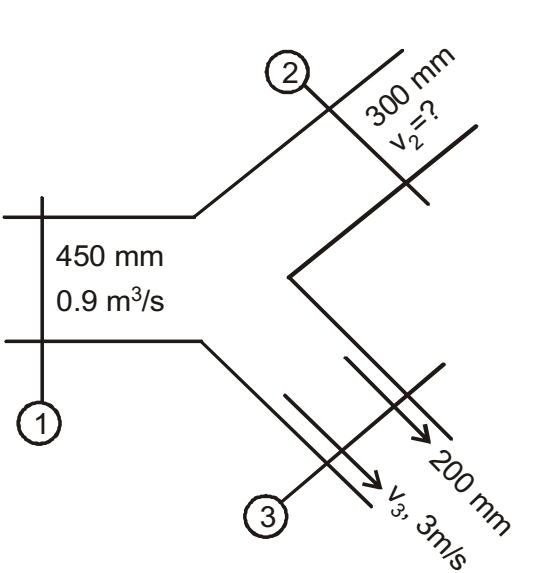Given data:
b1 = 450 mm = 0.45 m
b2 = 0.3 m
b3 = 0.2 m
Depth: h = 1m
Q1 = 0.9 m3 /s
V3 = 3m/s
V2 =?
Q1 = Q2 + Q3
Q1 = A2 V2 + A3 V3
Q1 = b2 hV2 + b3 hV3
0.9 = 0.3 × 1 × V2 + 0.2 × 1 × 3 0.9 = 0.3 V2 + 0.6
V2 = 1 m/s

##### Correct Option: AGiven data:
b1 = 450 mm = 0.45 m
b2 = 0.3 m
b3 = 0.2 m
Depth: h = 1m
Q1 = 0.9 m3 /s
V3 = 3m/s
V2 =?
Q1 = Q2 + Q3
Q1 = A2 V2 + A3 V3
Q1 = b2 hV2 + b3 hV3
0.9 = 0.3 × 1 × V2 + 0.2 × 1 × 3 0.9 = 0.3 V2 + 0.6
V2 = 1 m/s

1. For steady flow of a vlscous In compressible fluid through pipe of constant of diameter, the average veloolty In the fully developed region is constant, Which one of the following statements about the average velocity in the developing region is TRUE?

1. It is constant and is equal to the average velocity in the fully developed region,

##### Correct Option: B

It is constant and is equal to the average velocity in the fully developed region,